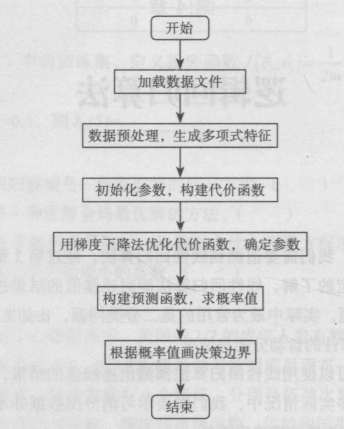## Python机器学习算法之逻辑回归算法

2021/4/19 23:34:10 文章标签:

• 1.算法概述
• 2.应用领域
• 3.算法步骤
• 4.算法实现
• 5.算法优化

## 1.算法概述

• 线性回归算法能对连续值的结果进行预测，而现实生活中最常见的还有分类问题，最为常见的是二分类问题，比如：判断一个人是否生病、现在是白天还是黑夜。为了解决诸如此类场景的问题，逻辑回归算法就诞生了。
• 逻辑回归算法是一种广义的线性回归分析方法，其仅在线性回归算法的基础上，套用一个逻辑函数，从而对事件发生的概率进行预测。
• 逻辑回归算法常用于数据挖掘、疾病自动诊断、经济预测等领域。

## 2.应用领域

• `用于预测`

根据逻辑回归模型，通过历史数据的表现，预测未来结果发生的概率

• `用于判别`

实际上与预测有些类似，即通过预测结果发生的概率，实现对数据的判别与分类

• `用于寻求影响结果的因素`

该算法主要在流行病学中应用较多，比较常用的情况是探索某种疾病的危险因素，即影响因素分析，包括从多个可疑影响因素中筛选出具有显著影响的因素变量

## 3.算法步骤1. 加载数据文件

2. 数据预处理，生成多项式特征

如果是最简单的二分类问题由于只有一阶特征，决策边界为一条直线，可以不考虑此步骤

3. 初始化参数θ，构建代价函数J(θ)

逻辑回归算法主要是使用最大似然估计的方法来学习

4. 利用梯度下降法优化代价函数J(θ)，确定参数θ

5. 构建预测函数，求概率值

逻辑回归算法通过拟合一个逻辑函数，即sigmoid函数，将任意的输入映射到[0,1]内

6. 根据概率值画出决策边界

所谓决策边界，就是能够把样本正确分类的一条边界，主要有线性决策边界和非线性决策边界

## 4.算法实现

``````import numpy as np
from sklearn import datasets
from utils import normalize, train_test_split, accuracy_score
from utils import Plot

# 定义sigmoid函数
def sigmoid(x):
return 1.0 / (1.0 + np.exp(-x))

# 自定义逻辑回归算法类
class LogisticRegression():
# 初始化函数，定义梯度下降学习率、梯度下降迭代次数
def __init__(self, learning_rate=.1, n_iterations=4000):
self.learning_rate = learning_rate
self.n_iterations = n_iterations

# 初始化参数，参数范围为[-1/sqrt(N), 1/sqrt(N)]
def initialize_weights(self, n_features):
limit = np.sqrt(1 / n_features)
w = np.random.uniform(-limit, limit, (n_features, 1))
b = 0
self.w = np.insert(w, 0, b, axis=0)

# 定义训练函数
def fit(self, X, y):
m_samples, n_features = X.shape
self.initialize_weights(n_features)
# 为X增加一列特征x1，x1 = 0
X = np.insert(X, 0, 1, axis=1)
y = np.reshape(y, (m_samples, 1))

# 梯度训练n_iterations轮
for i in range(self.n_iterations):
h_x = X.dot(self.w)
y_pred = sigmoid(h_x)
self.w = self.w - self.learning_rate * w_grad

# 定义预测函数
def predict(self, X):
X = np.insert(X, 0, 1, axis=1)
h_x = X.dot(self.w)
y_pred = np.round(sigmoid(h_x))

return y_pred.astype(int)

if __name__ == "__main__":
# 加载数据集
X = normalize(data.data[data.target != 0])
y = data.target[data.target != 0]
y[y == 1] = 0
y[y == 2] = 1

X_train, X_test, y_train, y_test = train_test_split(X, y, test_size=0.33, seed=1)

clf = LogisticRegression()
clf.fit(X_train, y_train)
y_pred = clf.predict(X_test)
y_pred = np.reshape(y_pred, y_test.shape)

accuracy = accuracy_score(y_test, y_pred)
print("精度为:", accuracy)

# 将数据集进行降维处理并展示
Plot().plot_in_2d(X_test, y_pred, title="Logistic Regression", accuracy=accuracy)
``````

## 5.算法优化

• `改进欠拟合`

欠拟合问题之所以出现是因为特征维度过小，以至于假设函数不足以学习特征和标签之间的非线性关系，所以解决思路是增加特征向量维度。

• `改进分类和回归的精度`

逻辑回归算法分类和回归精度不高主要是因为数据特征有缺失或者特征空间很大，可以通过正则化来解决这个问题。

暂无相关的数据...